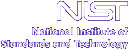Home FANS-HOME Instruments Contacts SiteMap

## Changing Monochromators

NOTE that though explained here, this procedure should be executed by a staff scientist (or under their supervision).

BT-4/FANS is equipped with two focussing monochromators designed to maintain high neutron intensity for all incident energies: Copper [Cu(220)], and Pyrolytic Graphite [PG(002)]. Note that the PG(002) monochromator suffers from l/2 contamination. Thus if one observes strong peaks at high energy transfer, these peaks will also appear at approximately E/4 in general. These spurious peaks are very sharp and weaker in comparison to the corresponding real features at higher energies. The monochromators are positioned such that the PG(002) is directly above the Cu(220). Since space is a valuable commodity inside the monochromator drum; there is very little room for divergence from the procedure that follows:

1. Drive the monochromator motor (motor #1) to a hardware angle of 25 degrees. This can be achieved by typing in icp: d1h=25
2. On the instrument rack immediately to the right of the computer, you will find the "goniometer drive" panel. This panel controls the X, Y, and Z motions of the monochromator among others. You will also find a table of values for each motion taped to the "goniometer drive" panel. Once step #1 above has been executed:
1. Turn the "goniometer drive" panel knob to the desired position to be moved
2. Place the "Drive" toggle in the direction of the motion (±)
3. Use the "Slew" switch to drive the monochromator to the desired position
4. Use the "Bump" switch when approaching the desired position to avoid overshooting
** OVERSHOOTING A MOTOR POSITION CAN DAMAGE THE MONOCHROMATOR **

1. Change the "software" value for the monochromator d-spacing i.e. set the proper d-spacing in icp). In icp type the command: dm=PG (or dm=Cu)

## Rocking Monochromators

BT-4/FANS requires a periodic adjustment of the motor #1 (monochromator) zero point correction to maximize the neutron flux incident on the detectors. In order to set the zero point, one must "rock the monochromator" at different incident energies and calculate the coefficients used in the correction. This can be accomplished by:

1. Driving the instrument to an incident energy using dem=[incident energy] and with the coupling flag TR- set.
2. Record the incident energy and θMONO value, i.e. thetaMONO = [angle], from the terminal window. This value of thetaMONO = [angle] is the angle necessary to meet the Bragg condition for the indicated incident energy position.
3. Search for the maximum neutron flux at the monitor by rocking motor #1.
 Command Description Terminal Window display fm1,2.,-.1,-2 Search for a peak using monitor counts. If peak found, can find a fit to the peak. The parameters are:  Monochromator motor. [2.] Range of peak search in degrees. [-.1] Search with negative steps in degrees. Necessary to avoid backlash throughout the scan. [-2] Time in seconds for each count. When search is done. Choose [y] for Gaussian fit. Angle no =1, Range =[38.084 ..36.084], Step = -0.100, Mon =-2 Angle01= 38.084 Intensity = 56 Angle01= 37.984 Intensity = 58 Angle01= 37.884 Intensity = 136 Angle01= 37.784 Intensity = 134 Angle01= 37.684 Intensity = 222 .................etc...................... Angle01= 36.284 Intensity = 43 Angle01= 36.184 Intensity = 27 Angle01= 36.084 Intensity = 16 Peak found at 37.253 with an intensity of 1092 Fit Results: BG = 36.346 Height = 1019.147 Pos = 37.2442 Width = -0.5794 Driving to fitted peak position!
4. Set the angle for motor #1 (the monochromator) to the thetaMONO = [angle] value found in step 2.
set1=[angle] Changes the angle of motor #1 (monochromator) and calculates the zero point correction.
5. Read the zero point values for the monochromator. This will reveal by how many degrees the monochromator was adjusted to meet the Bragg condition.
 Command Description Terminal Window display pz Displays the zero values for all motors Z01= 0.171 Z02= 0.433 Z03= 0.000 Z04= 3.060 Z05= 0.000Z06= 0.000 Z07= 0.000 Z08= -0.736 Z09= 0.000 Z10= 0.000Z11= 0.000 Z12= 0.000 Z13= 0.000 Z14= 0.000
6. Record the Z01 value in the log book. This is the zero correction value for motor #1 at the indicated incident energy.
7. Continue this process for various energies ( For example: E = 36.2, 50, 100.0, 140, and 160.) always recording the values in the log book.
8. The log book should have a table such as: (Remember that the values shown here are particular to this example. Your values will be different)
 E (meV) Z1 θB 36.2 0.421 36.024 50.0 0.412 30.028 100.0 0.386 20.723 140.0 0.384 17.401 160.0 0.385 16.245
Now using the data from the log book, calculate the slope and the intercept for θB versus Z1.1. Exit the Instrument Control Program (icp) by tying ex. (exiting the program is paramount, otherwise the changes made to the ZCOEFFS.CFG buffer below will not take effect) Change directory to /home/bt4/cfg and edit the configuration file (Be very careful not to alter any parameters other than the ones indicated here).
 Command Description Terminal Window display emacs ZCOEFFS.CFG Edits the configuration file ! z2 = z2coeff1 * A2 + z2coeff2 0.00316 !z2coeff1 0.31363 !z2coeff2 ! z1 = z1coeff1 * A1 + z1coeff2 0.007634 !z1coeff1 0.1510 !z1coeff2
Change the values for "slope" and "intercept" (ONLY FOR z1coeffs) to match the ones found in the fit. Be careful NOT to change any other value here. This will determine the optimal zero correction for the energy range used.
2. Save the ZCOEFFS.CFG buffer and return to the working directory (i.e.: [bt4@bt4 user]\$ ).
4. Set z1=-99. The -99 flag is used for a linear correction for the zero point versus theta. This step ensures that icp will use the zero point correction from the fit input in the ZCOEFFS.CFG buffer.
5. Verify that the proper flag was turned on as above. pz
6. If necessary, set the TR± flag.

Instrument scientists
 Craig M. Brown (301) 975 5134 Terry Udovic (301) 975 6241 Juscelino Leăo (301) 975 8867